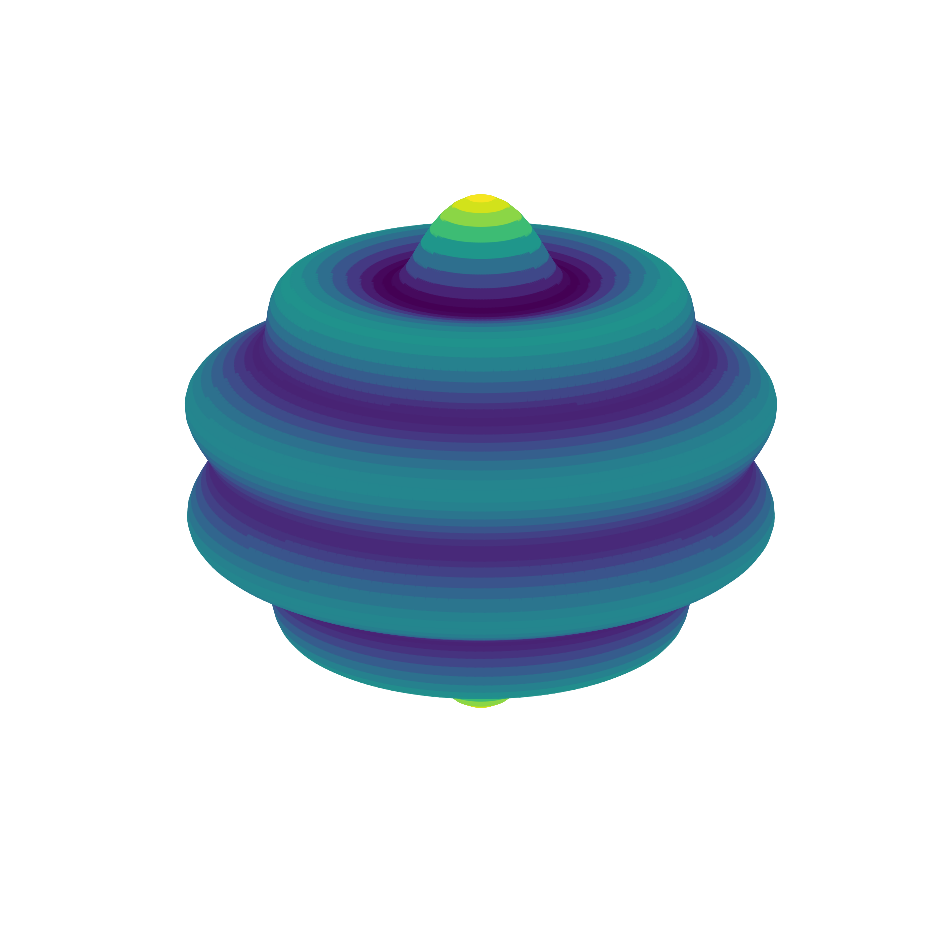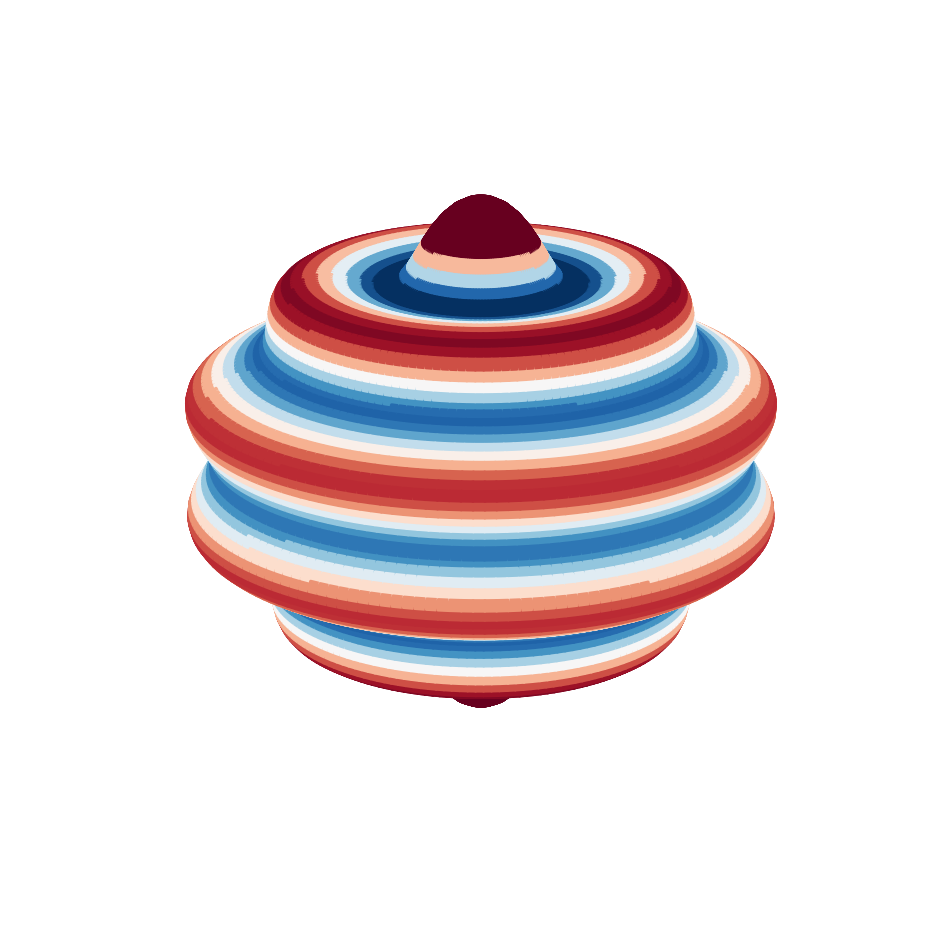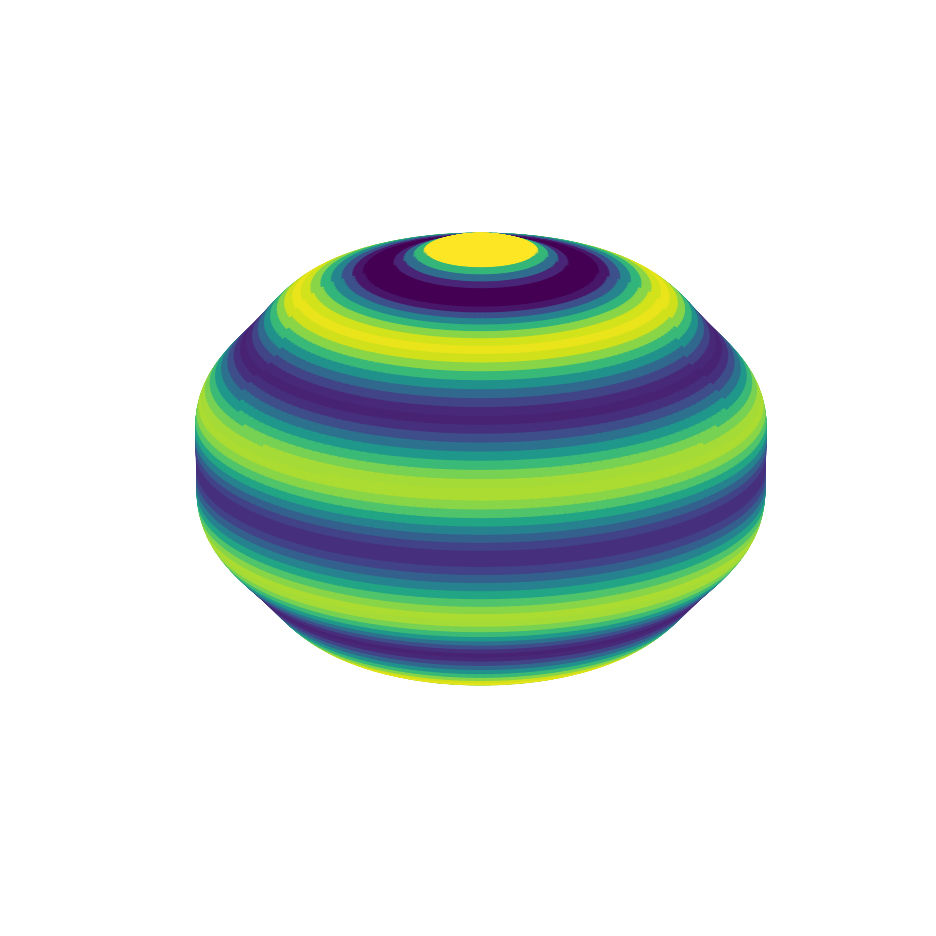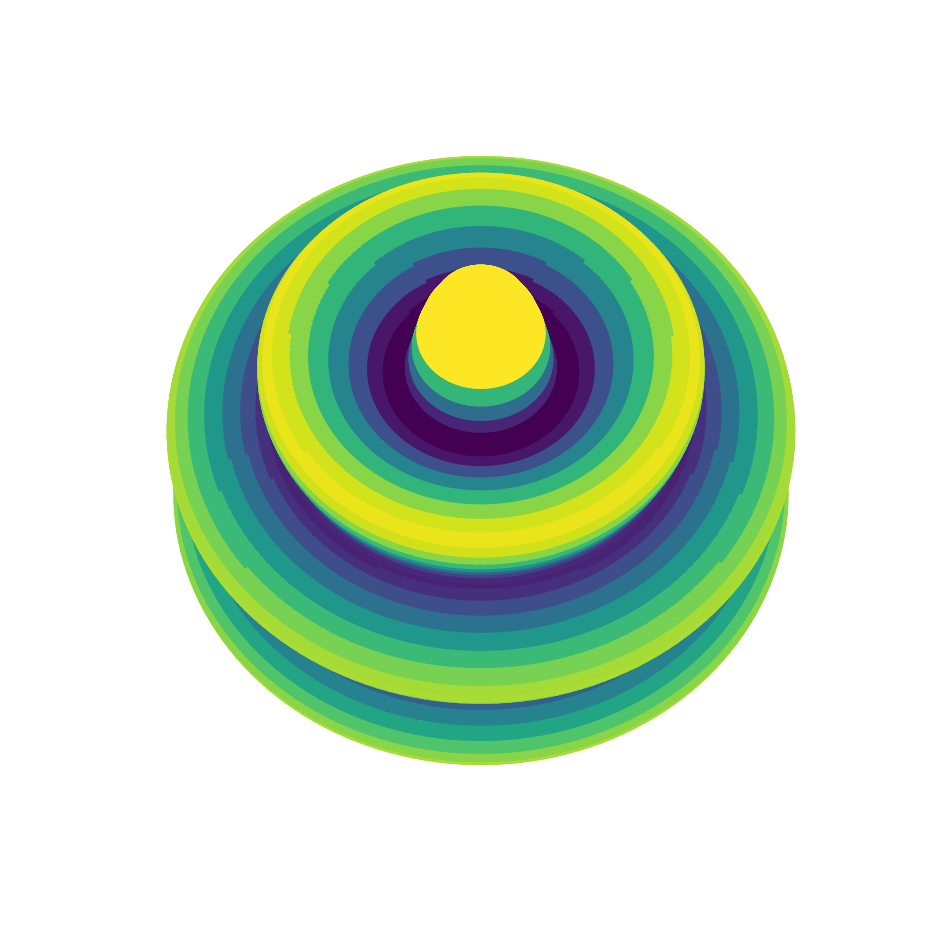# How to make 3-dimensional plots of gridded data¶

This example demonstrates how to plot a simple 3-dimensional representation of data stored in an SHGrid class instance using the method plot3d(). This plotting routine is useful for low resolution datasets, but becomes prohibitively slow when the spherical harmonic degree exceeds about 100.

In :
%matplotlib inline
import matplotlib.pyplot as plt
import pyshtools as pysh

pysh.utils.figstyle(rel_width=0.75)
%config InlineBackend.figure_format = 'retina'  # if you are not using a retina display, comment this line


We start by initializing a set of spherical harmonic coefficients to zero, and then set a single harmonic of degree 10 and order 0 equal to unity:

In :
lmax = 40  # This determines the spatial resolution of the gridded data and 3d image
clm = pysh.SHCoeffs.from_zeros(lmax)
clm.coeffs[0, 10, 0] = 1.
grid = clm.expand()


The plot3d() function first creates a 3-dimensional object from the data and then plots an image of the object from a given viewing geometry. The object is defined by its radius, which is computed from the data as

r = 1 + grid.data / abs(grid.data).max() / scale

As this equation shows, the gridded data are first normalized by their maximum value, they are then divided by a scaling factor, and the result is added to the unit sphere. For each point on the 3-dimensional object, the color correspond to the value of the original gridded data.

Here is what we get when we don't provide any optional arguments:

In :
fig, ax = grid.plot3d(show=False)    # show=False is used to avoid a warning when plotting in inline modeIt is important to note that the returned axes is a matplotlib Axes3dSubplot object, and not a standard AxesSubplot.

By default, plot3d() uses the color map 'viridis', but this can be modified by the parameter cmap. The color values are interpolated linearly from the minimum to maximum data values, and the minimum and maximum values can be changed by providing the parameter cmap_limits. In the followig plot, we choose to use the 'RdBu' colormap, and to limit the data values to the range of -1.5 to 1.5. Furthermore, we will reverse the sense of the colormap progression by specifying the cmap_reverse parameter:

In :
fig, ax = grid.plot3d(cmap='RdBu',
cmap_reverse=True,
cmap_limits=[-1.5, 1.5],
show=False)The optional scale parameter determines how much the radius of the object varies. The default value is 4: choosing a larger value will make the object look more like a sphere, whereas a smaller value will give more pronounced relief. Here we use a value of 20, which gives us more subtle wave-like features on a sphere:

In :
fig, ax = grid.plot3d(scale=20,
cmap_limits=[-1.5, 1.5],
show=False)The image can be viewed from any user specified elevation and azimuth. An elevation of 0° corresponds to looking directly at the equator, whereas 90° is looking at the north pole. The default values are 20° and 30°, respectively, and here we plot the data using a more poleward elevation of 60° instead.

In :
fig, ax = grid.plot3d(elevation=60,
azimuth=30,
scale=2,
cmap_limits=[-1.5, 1.5],
show=False)It is straightforward to insert 3d images in any matplotlib subplot. In this example, we use plt.subplots() to create a row of three axes, and the plot3d() function takes as an argument a single specified axes. Internally, this routine first removes the axes (which is a matplotlib AxesSubplot object), and then replaces it with an Axes3dSubplot object. In this example, we plot three different harmonics and add labels to each image using the title parameter.

In :
clm_tesseral = pysh.SHCoeffs.from_zeros(lmax)
clm_tesseral.coeffs[0, 10, 5] = 1.
grid_tesseral = clm_tesseral.expand()

clm_sectoral = pysh.SHCoeffs.from_zeros(lmax)
clm_sectoral.coeffs[0, 10, 10] = 1.
grid_sectoral = clm_sectoral.expand()

elevation = 0
azimuth = 150

fig, ax = plt.subplots(1,3, figsize=(10, 4))

grid.plot3d(elevation=elevation,
azimuth=azimuth,
cmap_limits=[-1.5, 1.5],
ax=ax,
title='Zonal\n$l=10, m=0$')
grid_tesseral.plot3d(elevation=elevation,
azimuth=azimuth,
cmap_limits=[-1.5, 1.5],
ax=ax,
title='Tessseral\n$l=10, m=5$')
grid_sectoral.plot3d(elevation=elevation,
azimuth=azimuth,
cmap_limits=[-1.5, 1.5],
ax=ax,
title='Sectoral\n$l=10, m=10$')
fig.tight_layout()